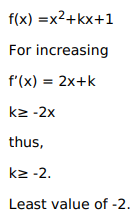# Solve this following

Question:

Mark $(\sqrt{)}$ against the correct answer in the following:

The least value of $k$ for which $f(x)=x^{2}+k x+1$ is increasing on $(1,2)$, is

A. $-2$

B. -1

C. 1

D. 2

Solution: